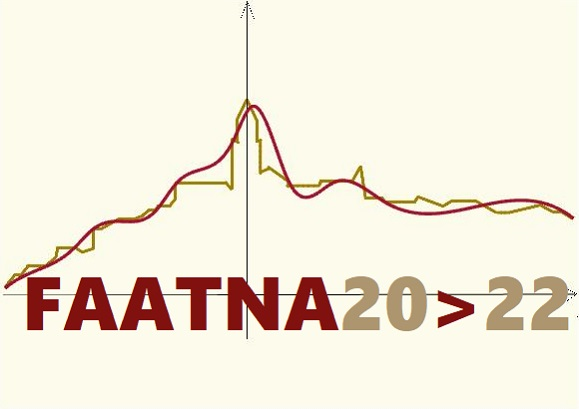# Functional Analysis, Approximation Theory and Numerical Analysis

## Matera, Italy, July 5-8, 2022

Session: Integral Equations: recent developments in numerics and applications

The aim of this special session is to present recent mathematical and computational developments on integral equations, as well as their applications. In particular, the talks will focus on integral equations with nonsmooth data defined on intervals, and on boundary integral equations associated with stationary or time-dependent PDE boundary value problems. Recent and efficient numerical approaches will be presented, together with their applications to different fields such as for example, acoustics, electromagnetics, heat conduction, elastodynamics.

Organizers:

• Luisa Fermo, fermo@unica.it
• Letizia Scuderi , letizia.scuderi@polito.it

Talks:

1. A. Aimi, Fast Energetic BEM for time-domain acoustic and elastic 2D scattering problems
2. M.C. De Bonis, Filtered interpolation and the numerical resolution of systems of hypersingular integro-differential equations
3. L. Desiderio, Energetic Boundary Element Method for 3D wavefield modelling
4. P. Díaz de Alba, An averaged Nyström method for 2D Fredholm integral equations
5. G. Di Credico, E-BEM for the resolution of 2D interior elastodynamic problems
6. S. Falletta, Coupling of curved virtual element with boundary element methods for exterior wave propagation problems
7. M. Ferrari, Recent results on the stability of the non-symmetric coupling of finite and boundary elements
8. C. Laurita, A stable BIE method for Laplace's equation with Neumann boundary conditions in domains with piecewise smooth boundaries
9. M. Lopez-Fernandez, New fast and oblivious convolution quadrature based on the global inversion of the Laplace transform
10. D. Mezzanotte, A numerical method for Volterra integral equations based on equispaced nodes
11. I. Notarangelo, "Truncated" Gaussian quadrature rules for exponential weights
12. T. Okayama, Sinc-collocation methods with consistent collocation points for Fredholm integral equations of the second kind
13. T. Pötzsche, Numerical Dynamics of Integrodifference Equations: Invariant Manifolds
14. G. Rodriguez, Regularized minimal-norm solution of overdetermined first kind integral models
15. V. Sagaria, Numerical method for BVP problems on real line